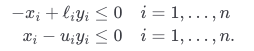•Gurobi Staff

The constraints $$\ell_i y_i \leq x_i \leq u_i y_i,\ i = 1, \ldots, n$$ can't be modeled as simple bound constraints, because the bounds are not constants. These constraints should be incorporated into the constraint matrix as

\begin{alignat*}{2} -x_i + \ell_i y_i &\leq 0 \quad && i = 1, \ldots, n \\ x_i - u_i y_i &\leq 0 && i = 1, \ldots, n.\end{alignat*}

If you're only trying to add $$[0, 1]$$ bounds to your variables for now, that is implemented correctly.

The $$\texttt{vtype}$$ field should be a one-dimensional vector of length $$2n$$. It is currently defined as a $$2 \times n$$ $$\texttt{char}$$ array:

>> [repmat('C',1,n) ; repmat('B',1,n)]ans =  2×10 char array    'CCCCCCCCCC'    'BBBBBBBBBB'

This will cause the variables types to not match what you intend. Instead, try:

model.vtype = [repmat('C',n,1); repmat('B',n,1)];

Note that you can use the gurobi_write() function to save the model as an LP file for visual inspection:

gurobi_write(model, 'model.lp');
•I've modified my model, but probably I wrote in a wrong way this new constraintsI 've used the vector [-eye(n) zeros(1,n)*eye(n) ; eye(n) -(ones(1,n)*eye(n) ]

while for the lower and upper bounds, respectively zeros(2*n,1) and ones(2*n,1)

Something is wrong

P.S thanks for your help, is the first time for me in this kind of optimization using Gurobi

•Gurobi Staff

The constraints

\begin{alignat*}{2} -x_i + \ell_i y_i &\leq 0 \quad && i = 1, \ldots, n \\ x_i - u_i y_i &\leq 0 && i = 1, \ldots, n.\end{alignat*}

can be equivalently written as

\begin{align*}\begin{bmatrix}-I &\phantom{-}L\\ \phantom{-}I &-U\end{bmatrix} \begin{bmatrix}x \\ y\end{bmatrix} &\leq \begin{bmatrix} {\bf{0}} \\ {\bf{0}} \end{bmatrix},\end{align*}

where $$I \in \mathbb{R}^{n \times n}$$ is the identity matrix, $$L \in \mathbb{R}^{n \times n}$$ is the diagonal matrix formed by the $$\ell_i$$, $$U \in \mathbb{R}^{n \times n}$$ is the diagonal matrix formed by the $$u_i$$, and $${\bf{0}} \in \mathbb{R}^n$$ is the vector of zeros.

You can add these constraints to the model by modifying the $$\texttt{A}$$, $$\texttt{sense}$$, and $$\texttt{rhs}$$ fields of your $$\texttt{model}$$ $$\texttt{struct}$$. Let's assume $$\ell_i = 0$$ and $$u_i = 1$$ for all $$i = 1, \ldots, n$$. Then $$L$$ is the matrix of zeros and $$U$$ is the identity matrix:

Aeq = [ones(1,n), zeros(1,n); zeros(1,n), ones(1,n); -eye(n), zeros(n); eye(n), -eye(n)];model.A = sparse(Aeq);model.rhs = [1; K; zeros(2*n,1)];model.sense = ['='; repmat('<',2*n+1,1)];

In this case, because $$\ell_i = 0$$ for all $$i = 1, \ldots, n$$ and $$x$$ is nonnegative, the constraints $$-x_i + \ell_i y_i \leq 0$$ are redundant. Thus, you can skip these constraints:

Aeq = [ones(1,n), zeros(1,n); zeros(1,n), ones(1,n); eye(n), -eye(n)];model.A = sparse(Aeq);model.rhs = [1; K; zeros(n,1)];model.sense = ['='; repmat('<',n+1,1)];